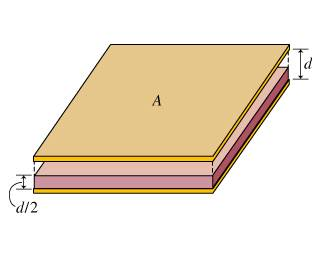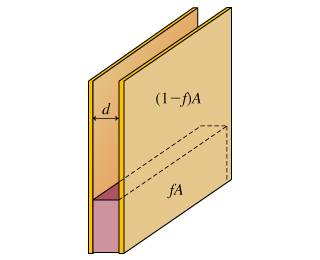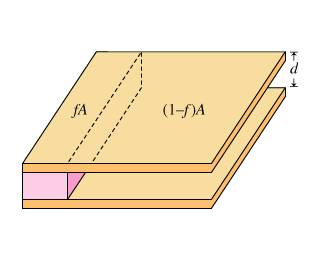# Capacitors with partial dielectrics

[SOLVED] Capacitors with partial dielectrics

## Homework Statement

Consider two parallel-plate capacitors identical in shape, one aligned so that the plates are horizontal, and the other with the plates vertical

horizontal:vertical:The horizontal capacitor is filled halfway with a material that has dielectric constant . What fraction of the area of the vertical capacitor should be filled (as shown in the figure) with the same dielectric so that the two capacitors have equal capacitance? Express your answer in terms of K

## Homework Equations

e = epsilon_0

Ch (horizontal capacitance) = 2KAe/d(K+1)

Cv (vertical capacitance) = Ae(1-f+Kf)/d

## The Attempt at a Solution

I've already found the capacitance of both capacitors, however it's asking for a fraction of f by itself, I've tried a few combinations, a ratio, an equivalence and I can't seem to cancel out enough variable to get f by itself in one side such that I have an answer in terms of K, if anybody could point me in the right direction, I would greatly appreciate it.

alphysicist
Homework Helper
Hi clope023,

They want Ch=Cv, so you can set the right hand sides equal to each other:

2KAe/d(K+1) = Ae(1-f+Kf)/d

and solve for f. When you solve for f, all of the other unknowns will cancel except for k.

Hi clope023,

They want Ch=Cv, so you can set the right hand sides equal to each other:

2KAe/d(K+1) = Ae(1-f+Kf)/d

and solve for f. When you solve for f, all of the other unknowns will cancel except for k.

hey thanks! got the answer, now I actually have another dielectric problem, I've more or less solved it utlizing the meaning of C, but they want the answer in terms of C as a function of f, let me show you:I know the total Capacitance = Cdieletric and Cempty

Cdielectric = KeAf/d

Cempty = eA(1-f)/d

C(f) = KeAf/d + eA(1-f)/d

my original idea was to write KC_0 + C_0(1-f), would this be the correct way of writing this equation as a function of f?

alphysicist
Homework Helper
I'm not certain what you are looking for here. You are missing an f in your last expression (needs to be

KC_0 f + C_0(1-f)

Did they just want an expression for the total capacitance with f in it? Or something else?

If you still have questions, could you post the entire problem?

I'm not certain what you are looking for here. You are missing an f in your last expression (needs to be

KC_0 f + C_0(1-f)

Did they just want an expression for the total capacitance with f in it? Or something else?

If you still have questions, could you post the entire problem?

they wanted Capacitance as a function of f, here's the question:

Consider a parallel-plate capacitor that is partially filled with a dielectric of dielectric constant K. The dielectric has the same same height as the separation of the plates of the capacitor but fills a fraction f of the area of the capacitor. The capacitance of the capacitor when the dielectric is completely removed is C_0, What is the capacitance C(f) of this capacitor as a function of f?

so as I said it was the Cdielectric + Cempty, so I think what you put will be correct, I'll check it.

it was correct, thanks alphysicist!

Hi clope023,

They want Ch=Cv, so you can set the right hand sides equal to each other:

2KAe/d(K+1) = Ae(1-f+Kf)/d

and solve for f. When you solve for f, all of the other unknowns will cancel except for k.

......I need help.....
My algebra seems to have left me; I can't get the right answer whilst trying to salve for f....

•suicidalbunny